# OpenCV/Python/dlib眨眼检测

+关注继续查看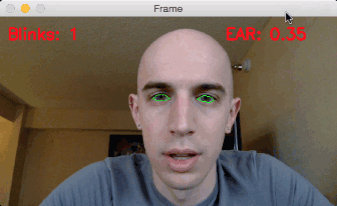OpenCVPythondlib进行眼睛眨眼检测

1.了解眼睛横比EAR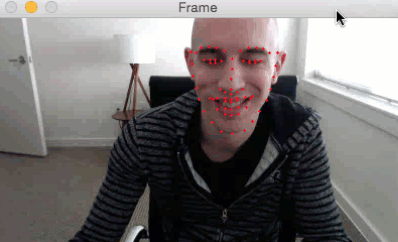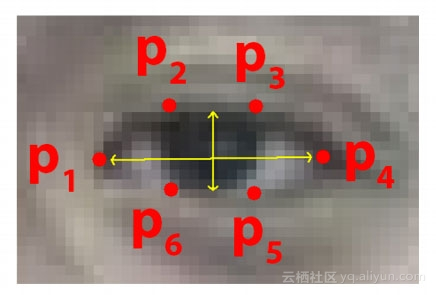SoukupováČech在其2016年的论文“使用面部标志实时眼睛眨眼检测的工作，我们可以推导出反映这种关系的方程，称为眼睛纵横比（EAR）：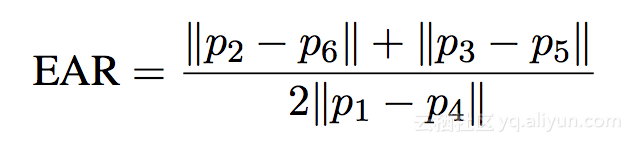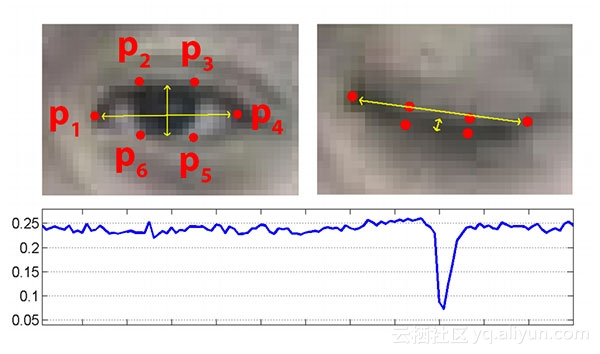2.用面部志和OpenCV检测眨眼（代码篇）

# import the necessary packages
from scipy.spatial import distance as dist
from imutils.video import FileVideoStream
from imutils.video import VideoStream
from imutils import face_utils
import numpy as np
import argparse
import imutils
import time
import dlib
import cv2


def eye_aspect_ratio(eye):
# compute the euclidean distances between the two sets of
# vertical eye landmarks (x, y)-coordinates
A = dist.euclidean(eye, eye)
B = dist.euclidean(eye, eye)
# compute the euclidean distance between the horizontal
# eye landmark (x, y)-coordinates
C = dist.euclidean(eye, eye)
# compute the eye aspect ratio
ear = (A + B) / (2.0 * C)
# return the eye aspect ratio
return ear


A，B是计算两组垂直眼睛标志之间的距离，而C是计算水平眼睛标志之间的距离。

# construct the argument parse and parse the arguments
ap = argparse.ArgumentParser()
help="path to facial landmark predictor")
help="path to input video file")
args = vars(ap.parse_args())


1.--shape-predictordlib预训练面部检测器的路径。

2.--video：它控制留在磁上的视频文件的路径。如果您想要使用实时视频流需在行脚本省略此开关。

# define two constants, one for the eye aspect ratio to indicate
# blink and then a second constant for the number of consecutive
# frames the eye must be below the threshold
EYE_AR_THRESH = 0.3
EYE_AR_CONSEC_FRAMES = 3
# initialize the frame counters and the total number of blinks
COUNTER = 0
TOTAL = 0


# initialize dlib's face detector (HOG-based) and then create
# the facial landmark predictor
detector = dlib.get_frontal_face_detector()
predictor = dlib.shape_predictor(args["shape_predictor"])


dlib库使用一个预先训练的人脸检测器，该检测器基于对用于对象检测的定向梯度直方图+线性SVM方法的修改。然后，我们初始化的实际面部标志预测值predictor。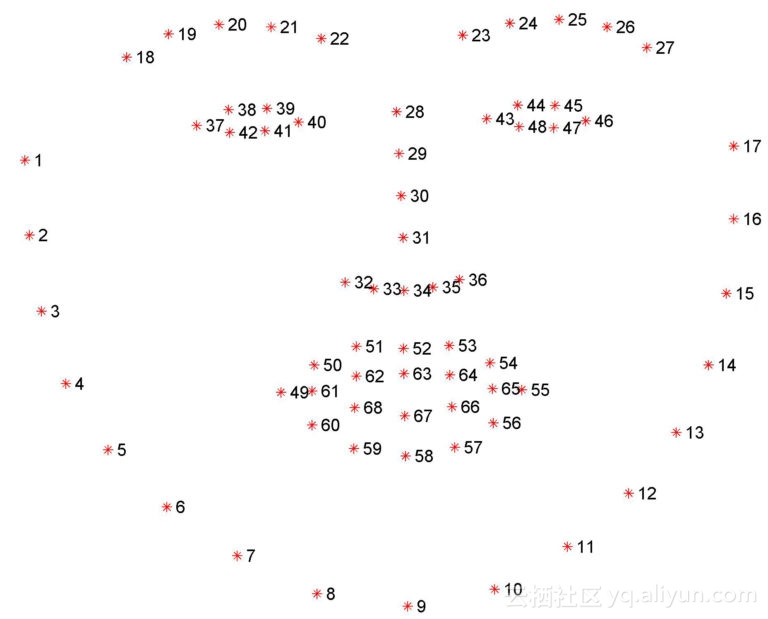# grab the indexes of the facial landmarks for the left and
# right eye, respectively
(lStart, lEnd) = face_utils.FACIAL_LANDMARKS_IDXS["left_eye"]
(rStart, rEnd) = face_utils.FACIAL_LANDMARKS_IDXS["right_eye"]


# start the video stream thread
vs = FileVideoStream(args["video"]).start()
fileStream = True
# vs = VideoStream(src=0).start()
# vs = VideoStream(usePiCamera=True).start()
# fileStream = False
time.sleep(1.0)


Raspberry Pi相机模块，取消注释：# vs = VideoStream(usePiCamera=True).start()

# loop over frames from the video stream
while True:
# if this is a file video stream, then we need to check if
# there any more frames left in the buffer to process
if fileStream and not vs.more():
break
# grab the frame from the threaded video file stream, resize
# it, and convert it to grayscale
# channels)
frame = imutils.resize(frame, width=450)
gray = cv2.cvtColor(frame, cv2.COLOR_BGR2GRAY)
# detect faces in the grayscale frame
rects = detector(gray, 0)


while我们开始从视频流循环帧。

# loop over the face detections
for rect in rects:
# determine the facial landmarks for the face region, then
# convert the facial landmark (x, y)-coordinates to a NumPy
# array
shape = predictor(gray, rect)
shape = face_utils.shape_to_np(shape)
# extract the left and right eye coordinates, then use the
# coordinates to compute the eye aspect ratio for both eyes
leftEye = shape[lStart:lEnd]
rightEye = shape[rStart:rEnd]
leftEAR = eye_aspect_ratio(leftEye)
rightEAR = eye_aspect_ratio(rightEye)
# average the eye aspect ratio together for both eyes
ear = (leftEAR + rightEAR) / 2.0


shape确定面部区域的面部标志，接着将这些（x，y）坐标转换成NumPy阵列。

# compute the convex hull for the left and right eye, then
# visualize each of the eyes
leftEyeHull = cv2.convexHull(leftEye)
rightEyeHull = cv2.convexHull(rightEye)
cv2.drawContours(frame, [leftEyeHull], -1, (0, 255, 0), 1)
cv2.drawContours(frame, [rightEyeHull], -1, (0, 255, 0), 1)


# check to see if the eye aspect ratio is below the blink
# threshold, and if so, increment the blink frame counter
if ear < EYE_AR_THRESH:
COUNTER += 1
# otherwise, the eye aspect ratio is not below the blink
# threshold
else:
# if the eyes were closed for a sufficient number of
# then increment the total number of blinks
if COUNTER >= EYE_AR_CONSEC_FRAMES:
TOTAL += 1
# reset the eye frame counter
COUNTER = 0


# draw the total number of blinks on the frame along with
# the computed eye aspect ratio for the frame
cv2.FONT_HERSHEY_SIMPLEX, 0.7, (0, 0, 255), 2)
cv2.putText(frame, "EAR: {:.2f}".format(ear), (300, 30),
cv2.FONT_HERSHEY_SIMPLEX, 0.7, (0, 0, 255), 2)
# show the frame
cv2.imshow("Frame", frame)
key = cv2.waitKey(1) & 0xFF
# if the q key was pressed, break from the loop
if key == ord("q"):
break
# do a bit of cleanup
cv2.destroyAllWindows()
vs.stop()


3.眨眼检测结

$python detect_blinks.py \ --shape-predictor shape_predictor_68_face_landmarks.dat \ --video blink_detection_demo.mp4 后来，在旅馆里，我记录下了眨眼检测器的实时流，并将其变成了屏幕录像。 要访问我的内置摄像头，我执行了下面的命令（注意取消注释正确的VideoStream类，如上所述）： $python detect_blinks.py \
--shape-predictor shape_predictor_68_face_landmarks.dat


4.的眨眼检测Opencv-Python学习笔记（二）
1610 0Opencv-Python学习笔记（一）
1738 0python-opencv学习笔记（二）
2558 0Python-OpenCV学习笔记（六）
1956 0Python-OpenCV学习笔记（五）
1847 0Python-OpenCV学习笔记（四）
1634 0python-opencv学习笔记（三）
2407 0python-opencv学习笔记（一）
1968 0Python 模块实战| 学习笔记

40 0python 模块argparse用法实例详解
1480 0
【方向】

706

5